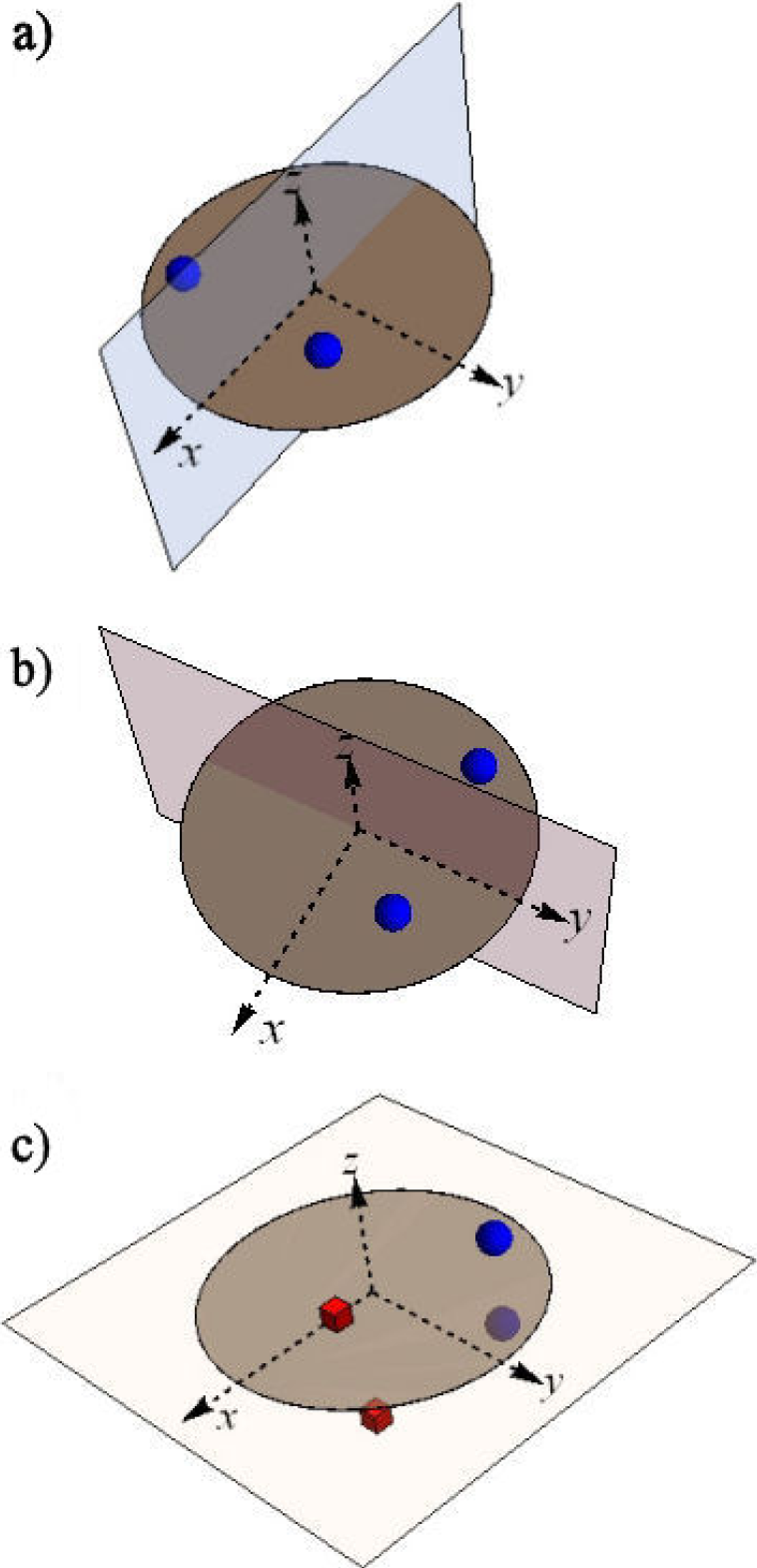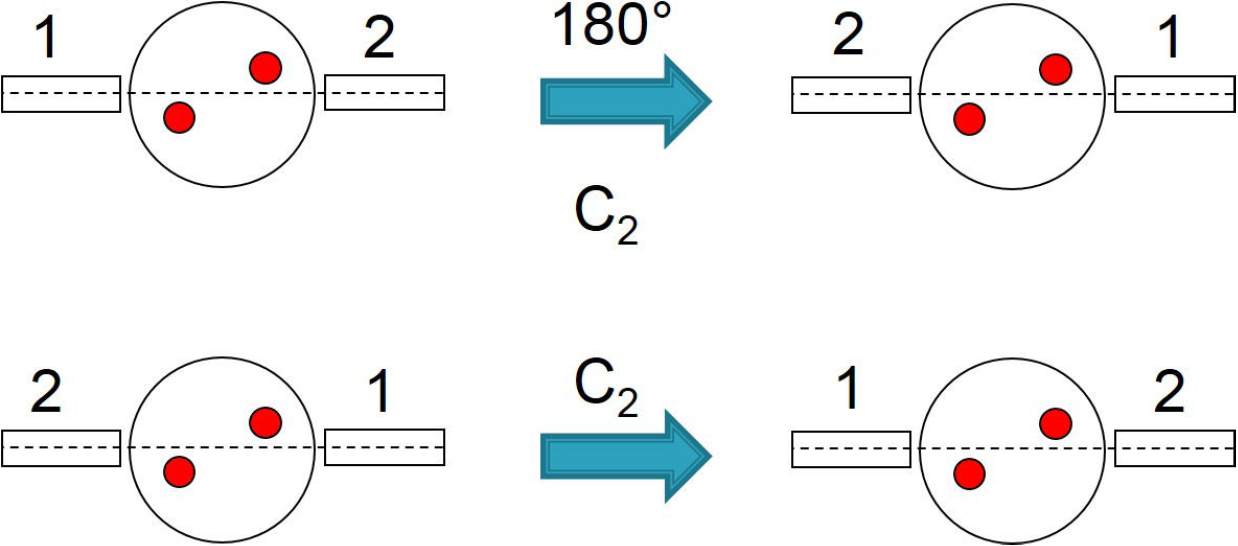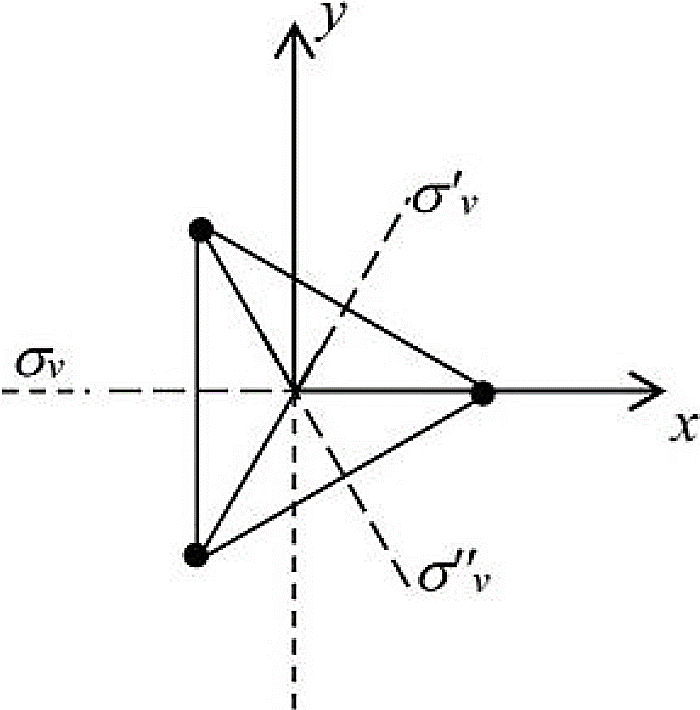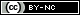## Articulo

•Citado por SciELO
•Accesos

•Similares en SciELO

## versión impresa ISSN 1870-3542

### Rev. mex. fís. E vol.62 no.1 México ene./jun. 2016

Education

About the calculation of the second-order susceptibility χ(2) tensorial elements for crystals using group theory

aCentro de Investigación en Ingeniería y Ciencias Aplicadas, Instituto de Investigación en Ciencias Básicas y Aplicadas, UAEM Cuernavaca, Mor. 62209, Mexico, * Catedrático CONACYT. e-mail: adalberto.alejo@uaem.mx

bTheoretical Physics Division, Department of Physics, Bogor Agricultural University, Jl. Meranti, Kampus IPB Darmaga, Bogor 16680, Indonesia.

cCenter for Surface- and Nanoanalytics, Johannes Kepler University, Altenbergerstr. 69, 4040 Linz, Austria.

ABSTRACT

In this work we discuss the way in which, in principle, the nonzero elements in the second-order susceptibility tensor are calculated in a crystal. Group Theory predicts which one of these elements will be zero based on the symmetry of the crystal. However, the position of these zeros in the tensor are intrinsically associated to a fixed system of reference chosen “a priori” for a particular crystal.

Keywords: Susceptibility; tensor; crystal; group theory

PACS: 02.20.-a; 42.65.An; 01.30.Bb

1. Introduction

Usually, studying nonlinear optics, one finds tables which have lists of the nonzero coefficients for the second-, third- and even fourth-order susceptibility tensors. A classic text book example regarding this topic is Nonlinear Optics by R. Boyd 1, where it is mentioned that the nonzero coefficients in the tables are calculated using group theory. However, there is no explanation there about the procedure to calculate these coefficients, the author says that such procedure is beyond the scope of the book and refer to the lector to more specialized books in this subject 2,3.

Mathematically, the optical nonlinear response is expressed as a relationship between the polarization P(ω) and the electric field E(ω) In the linear case, the polarization is simply expressed using a proportionality relation

P(ω)=χ(1)(ω)E(ω), (1)

where, the proportionality constant X(1), is the first-order susceptibility and it is an scalar (times the identity tensor), whereas the polarization and the electric field are vectors.

Instead, P(ω) in the nonlinear case can be modeled as a power series of the field E(ω) and is writing in a short form as:

P(ω)=χ(1)(ω)E(ω)+χ(2)(ω)E2(ω)+χ(3)(ω)E3(ω)+ (2)

The quantities X(2) and X(3) are known as the second- and third-order susceptibilities, respectively. In contrast, when the electric field is described in a full vectorial way, then in general X(1), X(2) and X(3) are the second, third and fourth rank tensors, respectively.

In the vector formalism, we can write the nonlinear second-order polarization as

P(ω)=χ(1)E(ω)+χ(2)(E(ω)E(ω))+χ(3)(E(ω)E(ω)E(ω))+ (3)

or writing the last equation in terms of the components

Pi=χij(1)Ej+χijk(2)EjEk+χijkl(3)EjEkEl+ (4)

So the second term in the above equation will be extensively discussed in this manuscript. It is the second-order nonlinear susceptibility and is a third-rank tensor denoted by three subscripts “i”, “j” and “k”.

In order to describe the procedure that is required to calculate the nonzero coefficients in the X(2) tensor for a given set of symmetry operations, a revision of basic concepts is necessary. Therefore, we present in Sec. 2 a brief review of the rotation matrix and also mirror and inversion matrices, with respect to a chosen plane and to one point, respectively. Section 3 discusses the theoretical foundation of group theory. Last part, Sec. 4, explains the procedure to determine the nonzero elements in X(2) for a particular system of reference using group theory. Furthermore, we discuss how to rotate a tensor a general angle around an arbitrary Cartesian axis of rotation, comparing the number of nonzero elements in the tensor in a rotating system of reference with a fixed one. Finally, the conclusions are given.

2. Rotation, mirror and inversion matrices

The standard definition of a rotation matrix in three dimensions, is given by

Rz(ϕ)=cosϕsinϕ0-sinϕcosϕ0001 (5)

where the subscript in the matrix R means that the axis of rotation is the z direction and the quantity between parenthesis ϕ, is the angle of rotation. Most of the time, this is the chosen axis of rotation but there are some cases, where a different axis of rotation is used. We are going to give one example of the latter.

In general, according to the symmetry of the crystals and in order to keep the lattice unchanged after a rotation, there are only four possible angles (π, 2π/3, π/2 and π/3) and of course the identity 2π The reason is that the crystal must fill all the space with the periodic repetition of a fundamental building block, therefore only two-, three-, four- and six-fold axis of symmetry exist. However, in the case of molecules or other objects, additional angles of rotation are possible 3.

For a mirror plane, it is understood a reflection using a plane in the same way that a mirror works in real world. Mathematically, this can be expressed in the form of a matrix. For example, when the mirror plane is in the xz plane, it has the form

σx=1000-10001 (6)

The minus sign in the matrix element (σx )22 implies a reflection trough the x axis in two dimensions [where the last row and last column in Eq. (6) are inexistent] and in the case of three dimensions; it implies a reflection through the xz plane.

In the same way a mirror plane in the y axis will have, exactly the same form as the matrix shown in Eq. (6) but now the element (σy )11 is the one negative. As the reader may have guessed, for a mirror plane perpendicular to the z axis (in the plane xy), the element (σz )33 now becomes negative. Graphically, the geometrical meaning of the mirror planes is shown in Fig. 1. It is worth to note that for the latter case (Fig. 1 c)), there is not a mirror plane in the yz plane (σy ) because in one side there are cubes and in the other one spheres. As a rule of thumb, there is a mirror plane if whatever thing in one side of the plane exists exactly equal in the other side, as an image in a mirror.Figura 1 Different mirror planes. a) σx , b) σy and c) σz

An inversion matrix, is defined as the minus of the identity matrix. Also, this is the result of the combined action of the three matrices that described the mirror planes mentioned above. Physically, at least one of the two situations must exist for an object to have inversion symmetry. Either it has all the mirror planes simultaneously (see Fig. 2a)) or there is a “mirror image” directly diametrically opposed trough the origin but the individual mirror planes are not present, as depicted in Fig. 2b).Figura 2 Different cases where inversion is present. a) Inversion is composed by three perpendicular mirror planes, each one exist by itself. b) There is inversion but not the independent mirror planes.

In the next section we will discuss the way in which all these matrices are related to each other and with the nonzero coefficients in the susceptibility for a particular crystal or structure.

3. Fundamentals of group theory

Group theory is a branch of mathematics, it is a general theory that can be applied to many situations, physics and other fields of mathematics. In essence, there are three necessary properties that must be fulfilled by a set of matrices to form a group under a particular operation, in this case the usual product of matrices. Therefore, the product of any two elements of the group generates also an element of the same group. The identity element (E) is always in the group as well as the inverse element or the reciprocal element for each element of the group is also in the group. For example, if a matrix A is inside a group, must be also inside this group its inverse element denoted by A-1 in such a way that A · A-1 =E

Now we are going to discuss two particular examples of a rotation group: C2 and C3υ . In the first case, the explicit matrix is given by

C2=Rz(π)=-1000-10001, (7)

this is a rotation of 180° around the z-axis. Thus, the product of this element by itself generates C2C2=C22, which as it is well known, two times a successive rotation of 180° is a full rotation of 360° and the object returns to its original position. Therefore, C22=E, which is easy to prove doing directly the product of matrices. Also, from this fact we can deduce that C2 is its own inverse C2=C2-1. This kind of rotation can be represented geometrically in two dimensions as can be seen in Fig. 3, where the numbers 1 and 2 are only labels to follow the rotations but both sides are indistinguishable.Figure 3 After two successive rotations of 180° an object returns to its original position.

A more complicated example is the group C3υ , here the rotations are done by 120° and also there is an additional element, there are mirror planes. Mirror planes are labeled here with a sigma σi (i = 1, 2, 3), which means that a reflection around the dotted lines generates exactly the same object (See Fig. 4). These mirror planes are planes perpendicular to the plane of the figure and actually can be imagined as a two-dimensional object in a plane.Figure 4 Three successive rotations of 120° will generate the original configuration.

In a similar way, if we take three times C3, the result is the object in the original position as can be seen in Fig. 4. Mathematically this is C33=E, which implies that C32=C3-1. All this can be verified directly doing the product of matrices using Eq. (5) with ϕ = 2π/3. For the mirror planes, two times the same reflection brings back to the same object, this means σi σi = E. Moreover, different combinations of mirror plane products generate the remaining mirror planes and the product of a mirror plane for a rotating matrix C3 will give as a result another element of this group. One can construct a table with all the possible products of two elements of this group and verify that each result will be an element of the group.

We are not going to discuss further higher groups of symmetry but we are going to show how this is related to the calculation of the susceptibility tensors that describe the atomic crystals response in nonlinear optics interactions. Therefore, the next section is devoted to this topic.

4. Neumann’s principle and second rank tensor transformation

The relation between the matrices and the nonzero elements in the susceptibility tensors is given via the Neumann’s principle 2:

The symmetry elements of any physical property of a crystal must include the symmetry elements of the point group of the crystal.”

This means that, after applying a symmetry operation belonging to a particular crystal, a particular property of this should remain the same, for instance the tensorial elements of the susceptibility (which is a physical property) must fulfill this condition. In our case, depending on the crystal we can apply the rotations or mirror planes or other symmetry operation belonging to the point group of the crystal to the susceptibility tensor and after these transformations, Neumann’s principle implies that it must remain the same.

Let’s start with a third-rank tensor χ'lmn , which is nothing else that a set of numbers (coefficients) provided on a given coordinate system. When we now apply a symmetry operation to the crystal, mathematically this is described as a linear transform through the relation,

χijk=RilRjmRknχ'lmn, (8)

which mixes the original coefficients. In general, this is the way for transforming a third-rank tensor. Here Rab is a matrix and the subscripts i, j and k are running from 1 to 3 in stance of the x, y and z coordinates. However, physics/Neumann’s principle then requires:

χijk=RilRjmRknχlmn, (9)

and now Rab is a particular symmetry operation matrix in the group and the tensor after the transformation must be the same tensor which is going to be transformed, this is χijk = χlmn .This procedure generates a system of equations which must be solved in order to get the nonzero elements in the susceptibility tensor and also as there are more equations than variables, consistency must be checked.

A general third rank tensor can be represented as 4

d=d111d121d131d112d122d132d113d123d133d211d221d231d212d222d232d213d223d233d311d321d331d312d322d332d313d323d333 (10)

where dijk can be understood in the following way. First index “i” is related to the external column vector and determines a row in it, second index “j” and the third one “k” are associated with the columns and rows in the internal 3 x 3 matrices.

Now, we can calculate the resulting susceptibility tensor for a crystal with group of symmetry C2. To avoid extra complications and keep this as clear as possible, we are going to take only a two-dimensional crystal or this could be thought only as the surface of the crystal. For doing this we need to specify a system of coordinates, in particular a possibility is shown in Fig. 5, where to the left an example of a crystal with C2 symmetry is shown and to the right there is a possible unit cell with a fixed system of coordinates is displayed.Figure 5 Right, a two-dimensional crystal with C2 symmetry. Left, a possible unit cell with a particular system of reference.

Therefore, to calculate the susceptibility third-rank tensor for a crystal with C2 symmetry, Eq. (9) is applied, where Rab is now the matrix given in Eq. (5) and the third-rank tensor is given by Eq. (10). Thus, after contracting the matrices with the tensor to the right side of the Eq. (9) gives

d111d121d131d112d122d132d113d123d133d211d221d231d212d222d232d213d223d233d311d321d331d312d322d332d313d323d333=-d111-d121d131-d112-d122d132d113d123-d133-d211-d221d231-d212-d222d232d213d223-d233d311d321-d331d312d322-d332-d313-d323d333, (11)

this system of equations shown above is very easy but in general could be more complicated. It is straightforward to think that for an element to be the same as its negative implies that it must be zero. Therefore after solving it the final tensor is

XZ2(C2)=00d13100d132d113d123000d23100d232d213d2230d311d3210d312d322000d333 (12)

which, as mentioned before, it is the susceptibility third-rank tensor for a crystal with C2 symmetry and the subscript z means that the z direction is used as the twofold rotation axis. However, this form of tensor is different when compared with the one given in general group theory literature, e.g. Powell’s book 4, which is reproduced below:

χp(2)(C2)=0d1210d1210d1320d1320d2110d2310d2220d2310d2330d3210d3210d3320d3320, (13)

Clearly, this tensor is totally different to the one shown in Eq. (12) but for the number of nonzero tensorial elements. Additionally, Powell assumes symmetry along the tensor diagonal. The difference between both tensors has its origin in the different system of reference chosen to calculate the nonzero elements according to the symmetry of the crystal in that system. In our case, the symmetry group is the same as in Powell’s but the latter uses a rotation about the y-axis. Hence, the matrix of rotation used by Powell is the following

C2=Ry(π)=-10001000-1. (14)

After using this matrix with Eq. (9), yields

d111d121d131d112d122d132d113d123d133d211d221d231d212d222d232d213d223d233d311d321d331d312d322d332d313d323d333=-d111d121-d131d112-d122d132-d113d123-d133d211-d221d231-d212d222-d232d213-d223d233-d311d321-d331d312-d322d332-d313d323-d333, (15)

which immediately leads to Eq. (13), reproduced again below:

χy(2)(C2)=0d1210d1120d1320d1230d2110d2310d2220d2130d2330d3210d3120d3320d3230, (16)

where subscript y means that the y direction is used as the twofold rotation axis.

We now move our discussion around the C3υ symmetry group and explain how one can get both possibilities enlisted in the tables from classic books 1,2. Moreover, we will show how to obtain from one configuration the other one and a general disposition of the nonzero elements in the tensor.

In this case, the rotation matrix will be defined with the rotation axis around z-axis and from Eq. (5), we get

C3=Rz2π3=-12320-32-120001. (17)

Now, we are going to consider the configuration given in Fig. 6, where an object with C3υ symmetry is sketched. Of course, there are also crystals with this symmetry but just for pedagogical illustration we are going to use this simple two-dimensional object.Figure 6 Object with C3υ symmetry, in a fixed system of coordinates. συ is the principal mirror plane in x-axis.

In this case, there is a mirror plane over the x-axis, the matrix that described it is given by

σvx=1000-10001 (18)

The others mirror planes, labeled συ' and συ'' can be obtained by applying a rotation of 60° (clockwise and anticlockwise direction) to this “principal” mirror plane. Using this mirror plane matrix Eq. (18) with Eq. (9) and the general tensor in Eq. (10), yields

d111d121d131d112d122d132d113d123d133d211d221d231d212d222d232d213d223d233d311d321d331d312d322d332d313d323d333=d111-d121d131-d112d122-d132d113-d123d133-d211d221-d231d212-d222d232-d213d223-d233d311-d321-d331-d312d322-d332d313-d323d333, (19)

which is a trivial system of equations with solution

di=d1110d1310d1220d1130d1330d2210d2120d2320d2230d3110d3310d3220d3130d333 (20)

and again, using Eq. (9) but now with the C3υ matrix given in Eq. (17) and this “intermediate” tensor, yields

d1110d1310d1220d1130d1330d2210d2120d2320d2230d3110d3310d3220d3130d333=-18(d111+3[d122+d212+d221])38(-d111+d122-3d212+d221])14(d131+3d232)38(-d111+d122+d212-3d221)18(-3d111-d122+3[d212+d221])34(d131-d232)14(d113+3d223)34(d113-d223)-12d13338(-d111-3d122+d212+d221)18(-3d111+3[d122+d212]-d221)34(d131-d232)18(-3d111+3d122-d212+3d221)-38(3d111+d122+d212+d221)14(3d131+d232)34(d113-d223)14(3d113+d223)-32d13314(d311+3d322)34(3d311-d322)-12d33134(d311-d322)14(3d311+d322)-32d311-12d313-32d313d333 (21)

The above system of equations is harder to solve than the one in Eq. (19), still it has a solution and it is consistent with all the equations (there are more equations that variables). When solved, the final tensor has the form:

χz(2)(C3v,σx)=d1110d1310-d1110d113000-d1110-d1110d1310d1130d311000d311000d333 (22)

Again, this tensor is different to the one given by Powell 4, which is reproduced below (please note that in the book of Powell, the coefficient “2” multiplying the elements X112 and X121 is wrong):

χp(2)(C3v)=0-2d222d131-2d22200d13100-d222000d222d1310d1310d311000d311000d333 (23)

The discrepancy occurs because the system of reference used to derive the tensor has not been defined a priori. Here, in this paper, it is chosen the y-axis as a principal mirror plane, as illustrated in Fig. 7, where the matrix is given by

σvy=-100010001 (24)Figure 7 Object with C3υ symmetry, in a fixed system of coordinates. συ is the principal mirror plane in y-axis.

(As a side comment we mention that in solid state physics and especially X-ray diffraction each crystallographic group and its atoms are described in a given coordinate system, and afterwards the symmetry elements are deduced in this predefined coordinate system, see e.g. Ref. 5).

This time, using this matrix Eq. (24) and the rotationmatrix given in Eq. (17) as well as the procedure described above, together with Eq. (9), yields the correct tensor for the system of reference shown in Fig. 7:

χz(2)(C3v,σx)=d1110d1310-d1110d113000-d1110-d1110d1310d1130d311000d311000d333 (25)

The tensor given in Eq. (22) and the last one obtained in Eq. (25) are related and as mentioned before the position of the nonzero elements in the tensor is going to change in accordance with the system of reference used to derive the nonzero independent elements in the tensor in agreement with group theory. To show this, we are going to use Eq. (8) again and transform the tensor given by Eq. (22) to a general rotating frame using the matrix from Eq. (5). Thus, after contracting the tensor, yields

χz(2)(C3v,Rz(ϕ))=d111cos(3ϕ)d111sin(3ϕ)d131d111sin(3ϕ)-d111cos(3ϕ)0d11300d111sin(3ϕ)-d111cos(3ϕ)0-d111cos(3ϕ)-d111sin(3ϕ)d1310d1130d311000d311000d333 (26)

Here, it is clear that the number of nonzero elements is bigger than before but still the number of independent elements remains the same. Moreover, when ϕ = 0the original tensor is recovered [Eq. (22)] and for the case of ϕ = π/2 the tensor given in Eq. (25) will be obtained only changing d111d222. Therefore, somehow the tensor still has some “memory” of the fixed system of reference used to calculate the nonzero elements due to its intrinsic symmetry.

For additional examples and discussion regarding the interaction of these tensors with the electromagnetic fields, the interested reader can consult Refs. 6 and 7.

5. Conclusions

The number of nonzero elements in a tensor associate to the susceptibility in a crystal strongly depends on the original system of reference in which the symmetry elements were defined. However, even if the relative position of the nonzero tensor elements change, the number of independent elements remains the same under any transformation by a rotation matrix defined by an arbitrary angle ϕ.

REFERENCES

1. R.W. Boyd, Nonlinear Optics, 2nd ed. (Academic Press, San Diego, 2003). [ Links ]

2. J.F. Nye, Physical Properties of Crystals, Their Representations by Tensors and Matrices (Clarendon, Oxford, 1957). [ Links ]

3. D.C. Harris and M.D. Bertolucci, Symmetry and Spectroscopy - An Introduction to Vibrational and Electronic Spectroscopy (Dover, New York, 1989). [ Links ]

4. R.C. Powell, Symmetry, Group Theory, and the Physical Properties of Crystals (Lecture Notes in Physics) (Springer, New York, 2010). [ Links ]

5. C.P. Brock (Editor-in-chief), International Tables for Crystallography (John Wiley and Sons, New York, 2014). Also, available online http://it.iucr.org/Links ]

6. A. Alejo-Molina, H. Hardhienata, and K. Hingerl, J. Opt. Soc. Am. B 31 (2014) 526. [ Links ]

7. A. Alejo-Molina , K. Hingerl , andH. Hardhienata , J. Opt. Soc. Am. B 32 (2015) 562. [ Links ]

Appendix

The contraction of tensors can be done easily using a commercial software as for example Mathematica®. Still, this is a little tricky because, this software only contracts the last index from the tensor to the left with the first index on the tensor to the right, from Mathematica’s help and documentation:

a, b gives an explicit result when a and b are lists with appropriate dimensions. It contracts the last index in α with the first index in b.”

Therefore, for doing the transformation given by Eq. (8), the command used is:

FullSimplify[Transpose[R.Transpose[R.d.Transporte[R],{2,1,3}],{2,1,3}]] (A.1)

where, R is a matrix of the symmetry group for the crystal under study, d is the general third-rank tensor from Eq. (10) and “Transpose” command interchange the indices with respect to the original order {1,2,3}.

Now, let’s explain how Eq. (A1) works. The procedure is a little bit complicated because the restriction in the contraction of the indices. First, we start from Eq. (8) again

Sijk=RilRjmRknSlmn' (A.2)

and transposing the last matrix Rkn and contracting also the first one, yields

Sijk=RilRjmTranspose[Rkn]Slmn'=RjmRilSlmn'Rnk=RjmSimk. (A.3)

After that, we can transpose the tensor, second index m to the first place i and contract with the last matrix, then one will have

Sijk=RjmTranspose[Simk,{2,1,3}]=RjmSmik=Sjik. (A.4)

Finally, we need to transpose back the tensor Sjik, interchanging again indices one (j) and two (i):

Sijk=Transpose[Sjik,{2,1,3}]=Sijk. (A.5)

The last step, is just to solve the system of equations and find the zeros or relations between the tensor elements. This procedure should be done again for every matrix in the symmetry group for the crystal under analysis.

Received: June 12, 2015; Accepted: August 31, 2015This is an open-access article distributed under the terms of the Creative Commons Attribution License